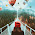## Wednesday, 13 January 2016

### Aggregate Fetch XML Calculation C# CRM

Hi Just want to share the Code to Calculate the Aggregate in CRM, especially for those who are in the CRM version, older than 2015 so that there will be no calculated and roll-up field so far.
Using this helper code you just need to pass the Parent Id, and it will do the rest of Calculation and of course you just need to supply the Operator (like SUM, Count, AVG, etc)

```public string CalculateAggregateFetchXML(string strEntityName, string strAggregateAttributeName,
AggregateOperator aggregateOperator, string strFilterXML) {
string strFetchXML = string.Empty;
string strAggregateAlias = "nec_alias";

strFetchXML = string.Format(@"
<fetch distinct='false' mapping='logical' aggregate='true'>
<entity name='{0}'>
<attribute name='{1}' alias='{2}' aggregate='{3}' />
{4}
</entity>
</fetch>", strEntityName, strAggregateAttributeName, strAggregateAlias, aggregateOperator.ToString(), strFilterXML);

EntityCollection aggregateResult = CrmService.RetrieveMultiple(new FetchExpression(strFetchXML));
decimal totalValue = 0;

foreach (var c in aggregateResult.Entities) {
decimal aggregate2 = 0;
if (c.Attributes.Contains(strAggregateAlias)) {
AliasedValue alias = ((AliasedValue)c[strAggregateAlias]);

if (alias.Value is Money) {
aggregate2 = ((Money)((AliasedValue)c[strAggregateAlias]).Value).Value;
} else if (alias.Value is Int32 || alias.Value is int) {
aggregate2 = ((int)((AliasedValue)c[strAggregateAlias]).Value);
} else if (alias.Value is Decimal || alias.Value is decimal) {
aggregate2 = ((decimal)((AliasedValue)c[strAggregateAlias]).Value);
}

totalValue = aggregate2;
}
}
}```

And the AggregateOperator Enum

```public enum AggregateOperator {
[Description("sum")]
sum = 1,
//
[Description("avg")]
avg = 2,
//
[Description("min")]
min = 3,
//
[Description("max")]
max = 4,
//
[Description("count(*)")]
count = 5,
//
[Description("countcolumn")]
countcolumn = 5,
}```

This usage is very easy

```strTotalAttByRace = CrmContext.CalculateAggregateFetchXML(“entityname” “primaryidfieldname”,
NEC.ESBU.Helpers.CrmHelper.AggregateOperator.count,strFilterXML);```

`//strFilterXML You can skip this or use this in case you need more condition`

It will result you a string or you can change to Integer, that is the total aggregate result

*Example for Aggregate Result with fetch xml

Is to return total Attendance by Race (that stored in the Contact entity)

Attendance is many to 1 relationship with Contact

```strFilterXML = string.Format(@"<filter type='and'>
<condition attribute='{0}' operator='eq' uitype='{1}' value='{2}' />
<condition attribute='pa_sessionid' operator='eq' uitype='pa_session' value='{3}' />
<condition attribute='pa_type' operator='eq' value='{4}' />
</filter>
<filter type='and'>
<condition attribute='pa_race' operator='eq' value='{5}' />
</filter>
strAttributeProductIdName, strEntityProductIdName, guidProductId.ToString(), guidSessionId.ToString(),
(int)productType, (int)raceType);```

This will be very useful if you want to calculate child record, either SUM, COUNT, or AVG for instance, then just pass the parent ID or add more fetch XML to add more filter

*Work for Money, Whole Number, and Decimal data type

Hope this helps!
Thanks

1.Nice! thanks!

2.Hi Aileen,
thanks for your work! it's great!
3.4.